# Development Aid and Climate Finance

## Abstract

This paper discusses the implications of climate change for official transfers from rich countries (the North) to poor countries (the South) when the motivation for transfers is ethical rather than strategic. Traditional development transfers to increase income and reduce poverty are complemented by new financial flows to reduce greenhouse gas emissions (mitigation transfers) and become climate-resilient (adaptation transfers). We find that in the absence of barriers to adaptation, mitigation or development, climate change will make isolated transfers less efficient: A large part of their intended effect (to increase income, reduce emissions, or boost climate-resilience) dissipates as the South reallocates its own resources to achieve the mitigation, adaptation and consumption balance it prefers. Only in the case of least-developed countries, which are unable to adapt fully due to income constraints, will adaptation support lead to more climate resilience. In all other cases, if the North wishes to change the balance between mitigation, adaptation and consumption it should structure its transfers as “matching grants”, which are tied to the South’s own level of funding. Alternatively, the North could provide an integrated “climate-compatible development” package that recognizes the combined climate and development requirements of the South. If the aim is to increase both mitigation and adaptation in the South, development assistance that increases the income level, can be an effective measure, but only if there is an international agreement and the recipient country is not income constrained. If the recipient country is very poor, development aid may reduce adaptation effort.

This is a preview of subscription content, access via your institution.

1. 1.

The Copenhagen Accord describes transfers that should “address the needs of developing countries”, see UNFCCC (2010).

2. 2.

There are examples of adaptation actions with regional public goods features, such as the management of international water systems, and global public good features, such as measures to deal with climate refugees (Heuson et al. 2012), but we can treat these as exceptions to the rule.

3. 3.

If mitigation and adaptation are complements, the poor region will boost its mitigation when it receives more adaptation support. This additional mitigation is beneficial for the rich region, given the public good nature of mitigation. So in that case, there are strong incentives for the rich region to voluntarily support adaptation in the poor region. Note that this argument is valid when there is no binding climate agreement.

4. 4.

Thus, we implicitly assume that real capital investments are made optimally.

5. 5.

For a discussion of the case where consumption and the environment are specified as two different goods in the utility function, see Hoel and Sterner (2007).

6. 6.

Buob and Stephan (2013) define mitigation and adaptation as substitutes if adaptation negatively affects the marginal productivity of mitigation. To see that this is the case in our model, we define a “green GDP function” as in Buob and Stephan; $$F(y,a,m)=y-\left[ {1-\alpha (a)} \right] D\left( {\sigma (m)y} \right) y$$. Based on this, the condition for substitution is fulfilled as $${\partial ^{2}F(y,a,m)} \big /{\partial m\partial a}=\alpha ^{\prime }D^{\prime }\sigma ^{\prime }y^{2}<0$$.

7. 7.

This is not important for the conclusions in this paper, but it simplifies the analyses as we get only one shadow price of carbon in the region, see Sect. 3 below.

8. 8.

Eyckmans et al. (2014) explore a global carbon constraint where carbon is traded between regions, thus introducing a strategic element into the game. Another alternative would be to associate the benefit of mitigation directly with reduced damage. This too would introduce strategic considerations into the model and make it difficult to distinguish the equity case for transfers from the strategic case. Moreover, our representation is not unrealistic. Very few countries are large enough to influence global emissions significantly. For most, the incentive to reduce emissions comes from an exogenously agreed target, rather than the possibility to reduce damage directly.

9. 9.

In reality, there will also be quick wins in improving adaptation capacity and productivity. At the same time, some mitigation efforts will only curb emission intensity in the long run. We abstract from these possibilities mainly because it allows us to keep the model tractable.

10. 10.

A linear utility function is of course a simplification. However, we may argue that transfers are not so big that they lead to large changes in the consumption levels of the regions, thus the marginal utility is relatively constant. With this representation, the marginal utility from a change in consumption is equal to one.

11. 11.

We could also introduce the inequality preferences in the welfare function of the South as in Kverndokk et al. (2014). This would give preferences for a higher consumption level in the South. However, as will be obvious from the discussions in Sects. 3 and 5 below, equity preferences will not affect the optimal mitigation and adaptation levels, and inequality aversion in the South would not matter for our analysis.

12. 12.

The general case would be $$U_N \left( {c_{N},c_{S}} \right) =c_N -\eta \max \left\{ {c_S -c_N ,0} \right\} -\mu \max \left\{ {c_N -c_S ,0} \right\} ,\quad c_j =c_j^0 +\delta _j c_j^1 ,\;\;j=N,S$$, where $$\eta$$ is a parameter representing the negative feeling of being worse off than the South, while $$\mu$$ is the parameter representing the negative feeling of being better off. We then have $$\eta \ge \mu$$. The second part of the welfare function equals zero as $$c_{N} >c_{S}$$.

13. 13.

We could also introduce a consumption transfer from North to South in both periods as a means to reduce consumption inequality. However, as we have assumed that $$\mu <1/2$$, no interior solution would be possible from the optimization problem, and there would not be any consumption transfer between the two regions. This is because utility is linear in consumption, and the North will always prefer one extra consumption unit to itself than to the South. A transfer is only welfare improving for the North if it increases consumption more in the South than it reduces consumption in the North. To be more precise:$$\Delta C_S >-\left[ {{\left[ {1-\mu } \right] } \big /\mu } \right] \Delta C_N$$. Note, however, that with a concave utility function, it may be welfare improving for the North to transfer consumption to the South if the consumption level is so high that the direct marginal utility of consumption (not considering inequality aversion) is very small. Thus, in this case we may find an optimal consumption transfer. Also, if $$\mu =0$$ so that North does not care about the welfare level of the South, there will not be any consumption transfer. The reason is that the consumption transfer has no strategic effect. The only reason to transfer consumption is that the North cares about the welfare of the South.

14. 14.

It seems likely that a global agreement would be heterogeneous. Some countries may have restrictions on absolute emission levels as in Eq. (1), while other countries may have restrictions on carbon intensity, i.e. $$\upsigma$$. If the latter is true, we have $$\sigma _j (m_j )=\bar{{\sigma }}_j$$. However, this will not change conclusions in the no-trade case as this also defines the mitigation level as a function of the exogenous target.

15. 15.

However, Bretschger and Suphaphiphat (2014) find that a mitigation transfer may be better for the South than development aid due to its effects on economic growth.

16. 16.

Note that this will not necessarily follow from the optimization problem of the North, see Sect. 5.

17. 17.

Again, this may not necessarily be an optimal policy for the North.

18. 18.

Note that there is an additional effect as the damage in period 1 is no longer given. However, for an individual country this effect will probably be negligible, see footnote 8.

19. 19.

In addition, higher income may lead to higher demand for climate protection, but we do not model this income effect.

20. 20.

We can do this because we have assumed that the marginal benefits of the first units of mitigation- and adaptation investments are unbounded.

21. 21.

Thus, as opposed to the result in the main model in Sect. 2, a consumption transfer may be optimal in this case.

22. 22.

Note that an adaptation transfer in this case would be equal to a pure consumption transfer. As discussed in footnote 13, a consumption transfer will not be optimal with a linear utility function. However, with a concave utility function we would get an interior solution, and it would be optimal with an adaptation transfer even if the adaptation level in the South did not increase. The reason is that consumption in South would increase and the inequality between the two sectors would be lower. This would increase the utility of the North as they express inequality aversion.

23. 23.

Note again that the transfer is only welfare improving, i.e., there exists an interior solution, if the cost of the transfer in the North (reduced consumption) is less than the increased consumption in the South that follows from the transfer.

24. 24.

Sufficient conditions for an interior solution are that the first unit of investment in adaptation has a very large effect on the residual damages $$(\lim _{a_j \rightarrow 0} {\upalpha }^{\prime }_j \left( {a_j } \right) =+\infty )$$ and that this effect vanishes for very large investments

$$(\lim _{a_i \rightarrow +\infty } {\upalpha }^{\prime }_i \left( {a_i } \right) =0)$$.

## References

1. Banerjee AV, Duflo E (2011) Poor economics: a radical rethinking of the way to fight global poverty. Public Affairs, New York

2. Barrett S (2003) Environment and statecraft. OUP, Oxford

3. Barrett S (2007) Why cooperate. The incentives to supply global public goods. OUP, Oxford

4. Barrett S (2008) Climate treaties and the imperative of enforcement. Oxf Rev Econ Policy 24:239–258

5. Bourguignon F, Sundberg M (2007) Aid effectiveness: opening the black box. Am Econ Rev 7(2):316–321

6. Bowen A, Fankhauser S (2011) The green growth narrative. Paradigm shift or just spin? Glob Environ Change 21:1157–1159

7. Bowen A, Cochrane S, Fankhauser S (2012) Climate change. Adapt Growth Clim Change 113(2):95–106

8. Bréchet T, Hritonenko N, Yatsenko Y (2013) Adaptation and mitigation in long-term climate policy. Environ Resour Econ 55:217–243

9. Bretschger L, Suphaphiphat N (2014) Effective climate policies in a dynamic North-South model. Eur Econ Rev 69:59–77

10. Buob S, Stephan G (2011) To mitigate or to adapt: How to confront global climate change. Eur J Polit Econ 27:1–16

11. Buob S, Stephan G (2013) On the incentive compatibility of funding adaptation. Clim Change Econ 4(2):1–18

12. Carraro C, Siniscalco D (1993) Strategies for the international protection of the environment. J Publ Econ 52(3):309–328

13. Carraro C, Eyckmans J, Finus M (2005) Exploring the full potential of transfers for the success of international environmental agreements, Note di Lavoro No $$>$$50, Fondazione ENI Enrico Mattei (FEEM), Milano

14. Chatterjee C, Sakoulis G, Turnovsky SJ (2003) Unilateral capital transfers, public investment, and economic growth. Eur Econ Rev 47:1077–1103

15. Collier P, Dollar D (2002) Aid allocation and poverty reduction. Eur Econ Rev 46(8):1475–1500

16. Collier P, Dollar D (2004) Development effectiveness: what have we learnt? Econ J 114(496):F244–F271

17. de Bruin K, Dellink R, Tol R (2009) AD-DICE: an implementation of adaptation in the DICE model. Clim Change 95:63–81

18. de Bruin K, Dellink R, Agrawala S (2009) Economic aspects of adaptation to climate change: integrated assessment modelling of adaptation costs and benefits. OECD environment working papers, No 6, OECD. doi:10.1787/225282538105

19. Dollar D, Easterly W (1999) The search for the key: aid, investment and policies in Africa. J Afr Econ 8(4):546–577

20. Easterly W, Pfutze T (2008) Where does the money go? Best and worst practices in foreign aid. J Econ Perspect 22(2):29–52

21. Ebert U, Welsch H (2012) Adaptation and mitigation in global pollution problems: economic impacts of productivity, sensitivity and adaptive capacity. Environ Resour Econ 52:49–64

22. Eyckmans J, Tulkens H (2003) Simulating coalitionally stable burden sharing agreements for the climate change problem. Resour Energy Econ 25:299–327

23. Eyckmans J, Fankhauser S, Kverndokk S (2014) Development aid and climate finance. Working paper 123, revised February 2014, Grantham Research Institute, London School of Economics

24. Fankhauser S (1994) The social costs of greenhouse gas emissions: an expected value approach. Energy J 15(2):157–184

25. Fankhauser S, Pearce DW (2014) Financing for sustainable development. In: Atkinson G, Dietz S, Neumayer E, Agarwala M (eds) Handbook of sustainable development. Edward Elgar, Cheltenham

26. Fehr E, Schmidt K (1999) A theory of fairness, competition, and cooperation. Quart J Econ 114(3):817–868

27. Grasso M (2010) An ethical approach to climate adaptation finance. Glob Environ Change 20:74–81

28. Haites E (ed) (2013) International climate finance. Routledge, London

29. Heuson C, Peters W, Schwarze R, Topp A-K (2012) Which mode of funding developing countries’ climate policies under the post-Kyoto framework? UFZ-Diskussionspapiere, No. 10/2012, Helmholtz Centre for Environmental Research (UFZ)

30. Hoel M (2001) International trade and the environment: How to handle carbon leakage. In: Folmer H, Landis Gabel H, Gerkin S, Rose A (eds) Frontiers of environmental economics. Edward Elgar, London

31. Hoel M, Sterner T (2007) Discounting and relative prices. Clim Change 84:265–280

32. Hong F, Karp L (2012) International environmental agreements with mixed strategies and investment. J Publ Econ 96(9–10):685–697

33. Ingham A, Ma J, Ulph AM (2005) Can adaptation and mitigation be complements? Working paper 79, Tyndall Centre for Climate Change Research

34. Ingham A, Ma J, Ulph A (2007) Climate change, mitigation and adaptation with uncertainty and learning. Energy Policy 35:5354–5369

35. Jacobs M (2013) Green growth. In: Falkner R (ed) Handbook of global climate and environmental policy. Wiley-Blackwell, Chicester

36. Kane S, Shogren JF (2000) Linking adaptation and mitigation in climate change policy. Clim Change 45:75–102

37. Kolstad C, Urama K, Broome J, Bruvoll A, Olvera M Cariño, Fullerton D, Gollier C, Hanemann WM, Hassan R, Jotzo F, Khan MR, Meyer L, Mundaca L (2014) Social, economic and ethical concepts and methods. In: Edenhofer O, Pichs-Madruga R, Sokona Y, Farahani E, Kadner S, Seyboth K, Adler A, Baum I, Brunner S, Eickemeier P, Kriemann B, Savolainen J, Schlömer S, von Stechow C, Zwickel T, Minx J (eds) Climate change 2014 mitigation of climate change. Contribution of Working Group III to the fifth assessment report of the Intergovernmental Panel on Climate Change. Cambridge University Press, Cambridge Chapter 3

38. Kverndokk S (1994) Coalitions and side payments in International $${\rm CO}_{2}$$ Treaties. In: van Ierland EC (ed) International environmental economics, theories and applications for climate change, acidification and international trade. Elsevier, Amsterdam, pp 45–74

39. Kverndokk S, Rose A (2008) Equity and justice in global warming policy. Int Rev Environ Resour Econ 2(2):135–176

40. Kverndokk S, Nævdal E, Nøstbakken L (2014) The trade-off between intra- and intergenerational equity in climate policy. Eur Econ Rev 69:40–58

41. Lee DS, Moretti E, Butler MJ (2004) Do voters affect or elect policies? Evidence from the US House. Q J Econ 119(3):807–859

42. Markandya A (1998) Poverty, income distribution and policy making. Environ Resour Econ 11(3–4):459–472

43. Markandya A (2002) Poverty alleviation, environment and sustainable development: implications for the management of natural capital. Discussion paper, The World Bank, Washington, DC

44. Markandya A (2008) Water and the rural poor: interventions for improving livelihoods in sub-Saharan Africa. FAO, Rome

45. Markandya A, Pearce DW (1991) Development, the environment, and the social rate of discount. World Bank Res Obs 6(2):137–152

46. Markandya A, Nurty MN (2004) Cost benefit analysis of cleaning Ganges: some emerging environment and development issues. Environ Dev 9(1):61–82

47. Nordhaus WD (2008) A question of balance: weighing the global warming policies. Yale University Press, New Haven

48. Pearce D, Barbier E, Markandya A (1990) Sustainable development. Economics and the environment in the third world. Earthscan, London

49. Pindyck R (2013) Climate change policy: What do the models tell us? NBER Working Paper No. 19244, July

50. Pittel K, Rübbelke D (2013a) International climate finance and its influence on fairness and policy. World Econ 36(4):419–436

51. Pittel K, Rübbelke D (2013b) Improving global public goods supply through conditional tranfers—the international adaptation transfer riddle, CESifo Working paper No. 4106

52. Sachs J (2005) The end of poverty: economic possibilities for our time. Penguin, New York

53. Schelling TC (1992) Some economics of global warming. Am Econ Rev 82(1):1–14

54. Schelling TC (1997) The cost of combating global warming: facing the tradeoffs. Foreign Aff 76(6):8–14

55. Stern N (2014a) Ethics, equity and the economics of climate change. Papers 1: science and philosophy. Econ Philos 30(3):397–444

56. Stern N (2014b) Ethics, equity and the economics of climate change. Papers 2: economics and politics. Econ Philos 30(3):445–501

57. Temple JRW (2010) Aid and conditionality. In: Rodrik D, Rosenzweig M (eds) Handbook of development economics, vol 5, Amsterdam, pp 4415–4523

58. Tol RSJ (2002) Estimates of the damage costs of climate change. Part II: dynamic estimates. Environ Resour Econ 21:135–160

59. Tol RSJ (2005) Emission abatement versus development as strategies to reduce vulnerability to climate change: an application of FUND. Environ Dev Econ 10:615–629

60. Tulkens H, van Steenberghe V (2009) Mitigation, adaptation, suffering. In search of the right mix in the face of climate change, CESifo working paper No. 2781

61. UNFCCC (2010) Report of the conference of the parties on its fifteenth session, held in Copenhagen from 7 to 19 December 2009. Addendum–part two: action taken by the conference of the parties, 30 March

62. World Bank (2010) World development report 2010. Development and climate change. Oxford University Press, Oxford

63. World Bank (2012) Inclusive green growth: the pathway to sustainable development. World Bank, Washington, DC

## Acknowledgments

The project was supported financially by the MILJØ2015 program at the Research Council of Norway. Fankhauser also acknowledges financial support by the Grantham Foundation for the Protection of the Environment and the UK Economic and Social Research Council. We are grateful for the discussions with Scott Barrett and comments from two anonymous referees, Katinka Holtsmark, Torben Mideksa, Linda Nøstbakken and various seminar participants. The authors are associated with CREE - the Oslo Centre for Research on Environmentally Friendly Energy—is supported by the Research Council of Norway.

## Author information

Authors

### Corresponding author

Correspondence to Sam Fankhauser.

## Appendices

### Financial Transfers and Mitigation in the South

The optimization problem of the South with respect to mitigation is given by maximizing the following Lagrangian,

\begin{aligned} {max}_{m_{S}} \;{\mathcal {L}}_{S}&= c_{S}^{0} +\delta _{S} c_{S}^{1} + \lambda _{S} \left[ {\hat{{e}}_{S} -e_{S}^{0} -e_{S}^{1}} \right] \nonumber \\&= y_{S}^{0} -m_{S} -a_{S} +\delta _{S} \left\{ {1-\left[ {1-\alpha _{S}(a_{S} +T^{a})} \right] D_{S} \left( {\hat{{e}}} \right) } \right\} \left[ {y_{S}^{1} + T^{i}} \right] \nonumber \\&+\,\lambda _{S} \left\{ {\hat{{e}}_{S} -\sigma _{S} \left( {m_{S} + T^{m}} \right) \left[ {y_{S}^{0} + y_{S}^{1} + T^{i}} \right] } \right\} \end{aligned}
(22)

where $$\lambda _{S}$$ is the shadow price on carbon. Assuming the emissions constraint is binding, the necessary first-order condition (FOC) for an interior solution is

\begin{aligned}&\frac{\partial {\mathcal {L}}_S }{\partial m_S }=-1-\hbox {}\lambda _S {\sigma }^{\prime }_S \left[ {y_S^{0} +y_S^1 +T^{i}} \right] =0 \nonumber \\&\quad \Rightarrow {}\quad -\,1/{\sigma }^{\prime }_S \left[ {y_S^{0} + y_S^1 +T^{i}} \right] =\lambda _S \end{aligned}
(23)

### Financial Transfers and Adaptation in the South

The maximization problem of the South with respect to adaptation is given by:

\begin{aligned} {max}_{a_{S}} {\mathcal {L}}_S&= c_S^{0} +\delta _S c_S^1 +\lambda _S \left\{ {\hat{{e}}_S -e_S^0 -e_S^1 } \right\} \nonumber \\&= y_S^0 -m_S -a_S +\delta _S \left\{ {\left[ {1-\left[ {1-\alpha _S \left( {a_S +T^{a}} \right) } \right] D_S \left( {\hat{{e}}} \right) } \right] \left[ {y_S^1 +T^{i}} \right] } \right\} \nonumber \\&+\,\lambda _S \left\{ {\hat{{e}}_S -\sigma _S \left( {m_S +T^{m}} \right) \left[ {y_S^0 +y_S^1 +T^{i}} \right] } \right\} \end{aligned}
(24)

The necessary first-order condition for a maximum (interior solutionFootnote 24) with respect to adaptation effort, $$a_{S}$$, is given by:

\begin{aligned} -1+\delta _S {\alpha }^{\prime }_S D_S \left[ {y_S^1 +T^i } \right] =0. \end{aligned}
(25)

### The Financial Transfer Decisions of the North

The optimization problem for the North can then be written as

\begin{aligned} {{\max }_{T^{a},T^{i},T^{m}}} {\mathcal {L}}_N&= \left[ {1-\mu } \right] \left[ {y_N^0 -m{ }_N-a_{N}-T^{a}-T^{i}-T^{m}} \right] +\mu \left[ {y_S^0 -m{ }_S-a_S } \right] \nonumber \\&+\left[ {1-\mu } \right] \delta _N y_N^1 \left[ {1-\left[ {1-\alpha _N \left( {a_N } \right) } \right] } \right] D_N \left( {\hat{{e}}} \right) \nonumber \\&+\mu \delta _S \left[ {y_S^1 +T^{i}} \right] \left[ {1-\left[ {1-\alpha _S \left( {a_S +T^{a}} \right) } \right] } \right] D_S \left( {\hat{{e}}} \right) \nonumber \\&+\lambda _N \left[ {\hat{{e}}_N -\sigma _N \left( {m_N } \right) \left[ {y_N^0 +y_N^1 } \right] } \right] , \end{aligned}
(26)

where $$\lambda _N$$ is the shadow price of carbon in the North. The Kuhn-Tucker conditions, where the choices of the South are taken as given, are

\begin{aligned} \frac{\partial {\mathcal {L}}_N }{\partial T^{a}}&= -\left[ {1-\mu } \right] +\mu \delta _S \left( {y_S^1 +T^{i}} \right) D_S \left( {\hat{{e}}} \right) {\alpha }^{\prime }_S \left( {\frac{\partial a_S }{\partial T^{a}}+1} \right) \le 0 \end{aligned}
(27)
\begin{aligned} \frac{\partial {\mathcal {L}}_N }{\partial T^{m}}&= -\left[ {1-\mu } \right] -\mu \left( {\frac{\partial m_S }{\partial T^{m}}} \right) \le 0 \end{aligned}
(28)
\begin{aligned} \frac{\partial {\mathcal {L}}_N }{\partial T^{i}}&= -\left[ {1-\mu } \right] -\mu \left[ {\frac{\partial m_S }{\partial T^{i}}+\frac{\partial a_S }{\partial T^{i}}} \right] \nonumber \\&+\,\mu \delta _S \left[ \left[ {1-\left[ {1-\alpha _S \left( {a_s+T^{a}} \right) } \right] } \right] D_S \left( {\hat{{e}}} \right) +\left[ {y_S^1 +T^{i}} \right] {\alpha }^{\prime }_S \frac{\partial a_S }{\partial T^{i}}D_S \left( {\hat{{e}}} \right) \right] \nonumber \\&\le 0, \end{aligned}
(29)

where equality holds for interior solutions of the respective endogenous variables.

### Appendix 2: Mitigation and Adaptation in an Income-Constrained South

Consider the case where the South is constrained in the sense that a minimal consumption level $${\underline{c}}$$ is required in every period, see the optimization problem in Eq. (16) and the first order conditions (FOCs) in Eqs. (17) and (18).

Combining the two FOCs above and totally differentiating yields:

\begin{aligned}&\delta _S {\alpha }^{\prime \prime }_S \left[ {da_S +dT^a } \right] D_S \left[ {y_S^1 +T^i } \right] +\delta _S {\alpha }^{\prime }_S {D}^{\prime }_S d\hat{{e}}\left[ {y_S^1 +T^i } \right] +\delta _S {\alpha }^{\prime }_S D_S dT^i \nonumber \\&\quad = -d\lambda _S {\sigma }^{\prime }_S \left[ {y_S^0 +y_S^1 +T^i } \right] -\lambda _S {\sigma }^{\prime \prime }_S \left[ {dm_S +dT^{m}} \right] \left[ {y_S^0 +y_S^1 +T^i } \right] -\lambda _S {\sigma }^{\prime }_S dT^{i}\qquad \end{aligned}
(30)

Combining this with the differentiated minimal consumption constraint and emission constraint, we can write the following system of three equations in three endogenous variables:

\begin{aligned} \left\{ {\begin{array}{l} -da_S -dm_S =d{\underline{c}} \\ {\sigma }^{\prime }_S \left[ {y_S^0 +y_S^1 +T^i } \right] \left[ {dm_S +dT^{m}} \right] +\sigma _S dT^{i}=d\hat{{e}}_S \\ \delta _S {\alpha }^{\prime \prime }_S \left[ {da_S +dT^a } \right] D_S \left[ {y_S^1 +T^i } \right] +\delta _S {\alpha }^{\prime }_S {D}^{\prime }_S d\hat{{e}}\left[ {y_S^1 +T^i } \right] +\delta _S {\alpha }^{\prime }_S D_S dT^i \\ \quad = -d\lambda _S {\sigma }^{\prime }_S \left[ {y_S^0 +y_S^1 +T^i } \right] -\lambda _S {\sigma }^{\prime \prime }_S \left[ {dm_S +dT^{m}} \right] \left[ {y_S^0 +y_S^1 +T^i } \right] -\lambda _S {\sigma }^{\prime }_S dT^{i} \\ \end{array}} \right. \end{aligned}
(31)

Reorganizing and defining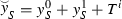and $$\bar{{y}}_S =y_S^1 +T^i$$, we get: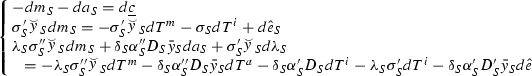(32)

Rewriting in matrix notation: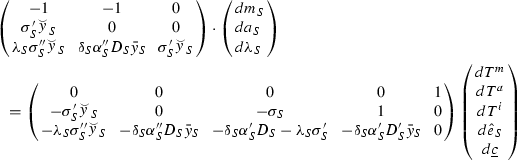(33)

It can easily be checked that the determinant of the coefficient matrix is positive and equal to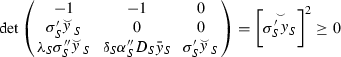(34)

The full comparative statics for mitigation are shown below.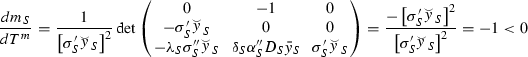(35)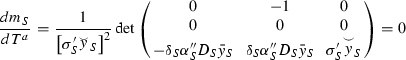(36)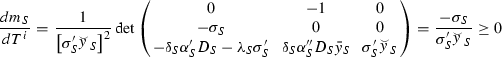(37)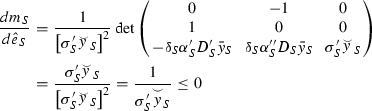(38)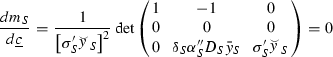(39)

The full comparative statics for adaptation are shown below.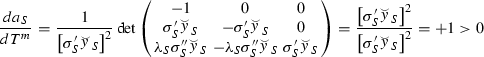(40)(41)(42)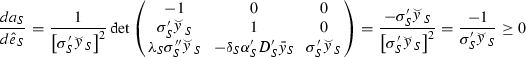(43)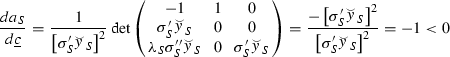(44)

## Rights and permissions

Reprints and Permissions